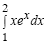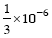# GATE | GATE CS 2008 | Question 21

• Last Updated : 16 Feb, 2018

The minimum number of equal length subintervals needed to approximateto an accuracy of at leastusing the trapezoidal rule is
(A) 1000 l
(B) 1000
(C) 100 l
(D) 100

Explanation: Trapezoidal rule error:Maximum error = 1/3 * 10^{-6} (given)

Therefore, |En| < 1/3 * 10^{-6}

a = 1 and b = 2 (given)

Therefore,f(x) = xe^x + 2e^x

f(x) is maximum at x=2.
Therefore, f(x) = 4e^2Thus, option (A) is correct.

Please comment below if you find anything wrong in the above post.

Quiz of this Question

My Personal Notes arrow_drop_up